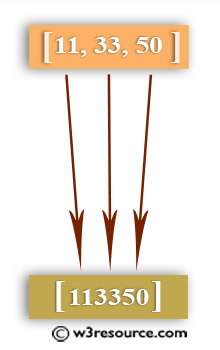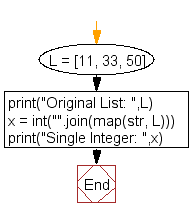﻿ Python: Convert a list of multiple integers into a single integer - w3resource

# Python: Convert a list of multiple integers into a single integer

## Python List: Exercise - 39 with Solution

Write a Python program to convert a list of multiple integers into a single integer.Sample Solution:-

Python Code:

``````L = [11, 33, 50]
print("Original List: ",L)
x = int("".join(map(str, L)))
print("Single Integer: ",x)
```
```

Sample Output:

```Original List:  [11, 33, 50]
Single Integer:  113350
```

Flowchart:## Visualize Python code execution:

The following tool visualize what the computer is doing step-by-step as it executes the said program:

Python Code Editor:

Have another way to solve this solution? Contribute your code (and comments) through Disqus.

What is the difficulty level of this exercise?

Test your Programming skills with w3resource's quiz.

﻿

## Python: Tips of the Day

Given a predicate function, fn, and a prop string, this curried function will then take an object to inspect by calling the property and passing it to the predicate:

Example:

```def tips_check_prop(fn, prop):
return lambda obj: fn(obj[prop])
check_age = tips_check_prop(lambda x: x >= 25, 'age')
user = {'name': 'Owen', 'age': 25}

print(check_age(user))
```

Output:

```True
```

We are closing our Disqus commenting system for some maintenanace issues. You may write to us at reach[at]yahoo[dot]com or visit us at Facebook